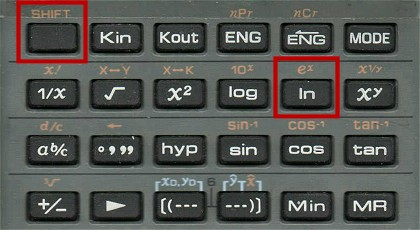Contents...

 >

 <

 Maths... Lesson 3: Euler's number
 Today I continued the section in my maths book on 'The exponential function' with the expression ex. It says: The most common exponential expression is ex where e is the exponential constant, 2.71828 . . . . Wikipedia says this: The number e, known as Euler's number, is a mathematical constant approximately equal to 2.71828 which can be characterized in many ways. It is the base of the natural logarithm. Euler is an interesting chap; he also has an asteroid named after him. Anyway, here is that function on my calculator:For e2 I do 2 [SHIFT] e2 = 7.389... I then worked through some examples in my maths book. I got somewhat stumped at trying to simplify these: e3y(1+ey) - e4y and (2et)2(3e-t) I then watched this video which I found to be good for understanding e. I followed along and used the Xy button on my calculator.